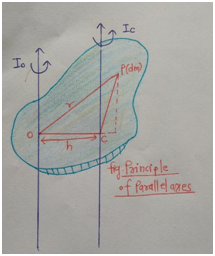# Parallel Axes Theorem

Theorem of Parallel axes gives the moment of inertia of a body about any axis in terms moment of inertia of a body about a axis parallel to first axis.

• We know that, the weight of the any body is due to its inertia. The moment of inertia of a body about any axis is nothing but the sum of product of mass of that each particle and square of the distance of each particle from that axis of rotation.
• That means, I = ∑ mi ri2

• The moment of inertia of the body depends on the mass, shape and size of the body.
• It also depends on the mass distribution of particles about the axis of rotation of the body.
• And also, on the position of the axis of rotation of the body.
• The SI unit of moment of inertia of the body is kg m2.

### Statement:

Theorem of Parallel axes states that the moment of inertia of a body about any axis is equal to the sum of moment of inertia about a axis parallel to it and passing through centre of mass of the body and the product of the mass of the body & square of the perpendicular distance between those two parallel axes.

The following is neat labelled diagram which explains the parallel axes theorem.• Thus, from figure we can write mathematical statement of parallel axes theorem as,

Io = Ic + Mh2

• Where, Io is the moment of inertia of a body about the axis passing through point O
• Ic is the moment of inertia of a body about the parallel axis passing through centre of mass C of the body.
• M is the total mass of the body
• And h is the perpendicular distance between the two parallel axis.

### Applications:

• Parallel axis theorem can be used to find the moment of inertia of about an axis in case of any body.
• Parallel axes theorem can be used to find moment of inertia of a thin rod.
• It is used to find moment of inertia of a ring about a tangent in its plane.
• It is also used to find the moment of inertia of ring about a tangent perpendicular to its plane.

#### Example:

What is the moment of inertia of a ring about a tangent in its plane?

Solution:Let Ic be the moment of inertia about the diameter of the ring as shown in figure.

And Io be the moment of inertia of a ring about the tangent which is parallel to diameter and the distance  between two parallel axis is h = R.

Then by parallel axes theorem,

Io = Ic + Mh2

We know that, the moment of inertia of a ring about its diameter is Ic = 1/2MR2

Thus, Io = 1/2MR2 + MR2

Io = 3/2 MR2

Thus, the moment of inertia of a ring about tangent in its plane is 3/2MR2.

Updated: April 11, 2022 — 3:07 pm